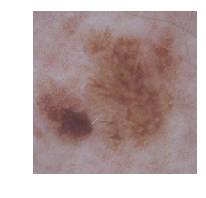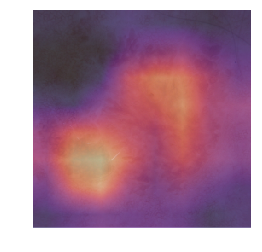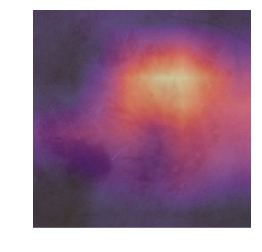# HAM10000 - CAM (class acitivated map)¶

## Skin Cancer MNIST: HAM10000 a large collection of multi-source dermatoscopic images of pigmented lesions¶

In :
%reload_ext autoreload
%matplotlib inline

In :
from fastai.vision import *
from fastai.metrics import accuracy

In :
PATH = "/home/katey/DeepLearning/Data/HAM10000/"

In :
label_csv = f'{PATH}HAM10000_metadata.csv'

In :
label_df = pd.read_csv(label_csv)


Note that perhaps a quarter of lesions have multiple images. We need to ensure that images from the same lesion do not appear in both the training and validation sets. Take a random sample of the unique lesion ids

In :
np.random.seed(827)
val_lesions = list(np.random.choice(label_df.lesion_id.unique(),size = 3000))

In :
val_idxs = label_df[label_df['lesion_id'].isin(val_lesions)].index


Make df with just the image-id and dx

In :
reduce_label_df = label_df.drop(columns = ['lesion_id','dx_type','age','sex','localization'])

In :
reduce_label_df.columns = ['filename','label']

In :
reduce_label_df.head()

Out:
filename label
0 ISIC_0027419 bkl
1 ISIC_0025030 bkl
2 ISIC_0026769 bkl
3 ISIC_0025661 bkl
4 ISIC_0031633 bkl
In :
label_csv = f'{PATH}labels.csv'
reduce_label_df.to_csv(label_csv, index = False)

In :
tfms = get_transforms(flip_vert = True)

In :
sz = 256
bs=32

In :
src = (ImageItemList.from_csv(PATH, 'labels.csv', folder = 'train', suffix = '.jpg')
.split_by_idx(val_idxs)
.label_from_df())

In :
data = (src.transform(tfms, size=sz)
.databunch(bs=bs).normalize(imagenet_stats))


Load previously trained model

In :
learn = create_cnn(data, models.resnet50, metrics=accuracy).load('mod-resnet50-sz256')


Pick which image to use from the validation set

In :
image_num = 87

In :
idx=image_num
x,y = data.valid_ds[idx]
x.show()
data.valid_ds.y[idx]

Out:
Category bkl## Heatmap¶

In :
m = learn.model.eval();


Create a minibatch with a single item, and put on the GPU

In :
xb,_ = data.one_item(x)
xb_im = Image(data.denorm(xb))
xb = xb.cuda()

In :
from fastai.callbacks.hooks import *


A 'hook' saves the output of the final layer of the convolutional part of the model, or m. The predictions are computed just to get the hook.

In :
def hooked_backward(cat=y):
with hook_output(m) as hook_a:
with hook_output(m, grad=True) as hook_g:
preds = m(xb)
preds[0,int(cat)].backward()
return hook_a,hook_g

In :
hook_a,hook_g = hooked_backward()

In :
acts  = hook_a.stored.cpu()
acts.shape

Out:
torch.Size([2048, 8, 8])

Compute the mean activations across the final layer; 2048 channels averaged, resulting in an 8 x 8 heatmap

In :
avg_acts = acts.mean(0)
avg_acts.shape

Out:
torch.Size([8, 8])
In :
def show_heatmap(hm):
_,ax = plt.subplots()
xb_im.show(ax)
ax.imshow(hm, alpha=0.6, extent=(0,256,256,0),
interpolation='bilinear', cmap='magma');

In :
show_heatmap(avg_acts)In :
grad = hook_g.stored.cpu()

(torch.Size([2048, 8, 8]), torch.Size())
mult = (acts*grad_chan[...,None,None]).mean(0)

show_heatmap(mult)